# Linear Regression using Excel

Link to video on Linear Regression using Excel

Regression Analysis is still the most popular method used in Predictive Analytics. The main reason is that it works. It is well known and understood. With its different flavors, regression analysis covers a width swath of problems. Another great reason to use it, is that regression tools are easy to find.

## What is Linear Regression?

Linear Regression is a method of statistical modeling where the value of a dependent variable based can be found calculated based on the value of one or more independent variables. The general idea, as seen in the picture below, is finding a line of best fit through the data. Using that line, you can then predict the value of Y given X.I am not going to go too deep into the math here. I highly the Khan Academy video posted below if you are looking to brush up on your statistics.

## Lets Start by Looking at the Data

If you download the Excel file at the top of the page, you will find 2 columns labeled Years and Salary. This example data set shows us the years of service and salary of 39 employees for an imaginary company.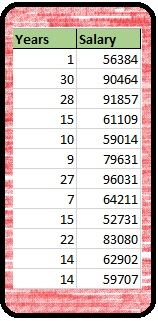What we are going to attempt to do is to develop a model using Linear Regression that will allow us to predict the salary of an employee given their years of service.

## Step 1: Build a Scatter Plot

The first thing we want to do is build a scatter plot. Excel makes this simple enough. Just highlight all of your data > select the Insert Tab from the Ribbon > Select Scatter from Charts: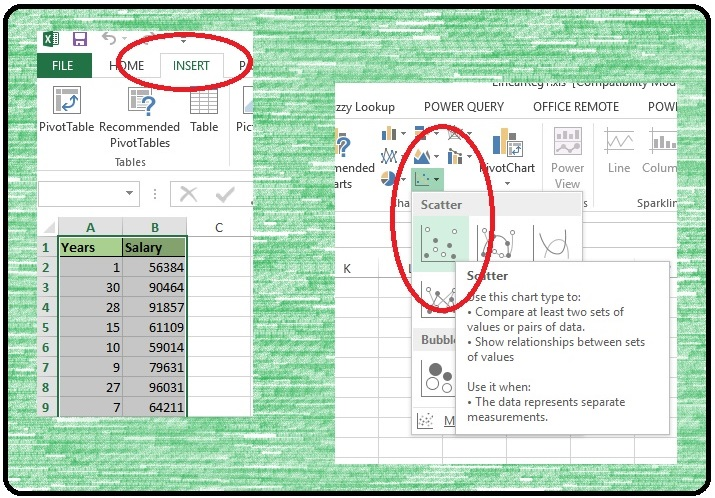What you will get should look something like this: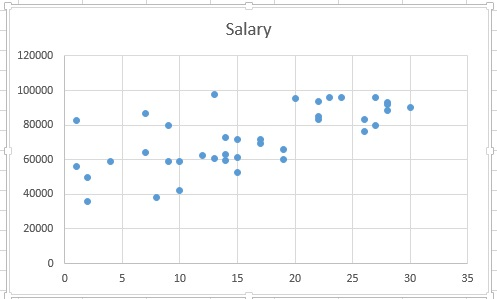We have a scatter chart with Salary on the Y Axis and Years on the X Axis. **Excel scatter charts set the left most column of the data set to the X Axis by default.

Before we move on, I want to take a moment to look at the scatter plot. Do you see a pattern? Can you see where you might be able to draw a line through the data?

I am not trying to just fill space here. I am asking a serious question. Because the answer is sometimes you will not see a pattern. Sometimes the scattering of data will be so random that there will no need to go forward with a linear regression. Learning to look for patterns in data visualizations is skill worth developing.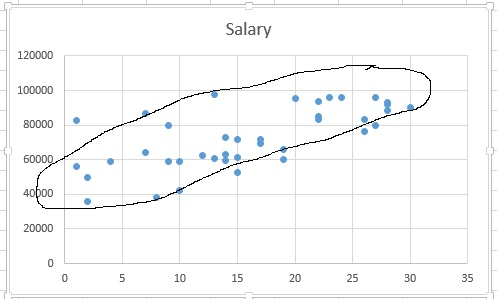In this example there is a general pattern, or more accurately, we see what looks like Positive Correlation. We call it positive because it appears that as X increases so does Y. So now that our scatter chart has passed the visual test, it is time perform our regression.

## Trend Line

Performing a simple linear regression in Excel is ridiculously easy. Simply click on your scatter plot > from the Ribbon select Chart Tools – Design > Add Chart Element > Trendline > LinearYour trendline appears on your chart. I personally find the line a little hard to see as is, so I am going to format it a bit.Start by double clicking on the trendline and the Format Trendline window will open on the right.

Line: — Color: Red  — Width: 3pt  — Dash type: Solid Line

Trendline Options — Select Display Equation on chart and Display R-squared value on chartAlright, that line is much easier to read. Now let us talk about the numbers in the circle. Now I know I said I was not going to get too deep into the math, but I feel I can’t do this subject justice without at least a cursory explanation of what is going on.What exactly did Excel do when it added the trendline? Technically it performed a statistical function known as Ordinary Least Squares.  What does that mean? Well if you wanted to attempt this by hand, one approach you could take would be to start by drawing a line that looked best to you. You would then measure the Residuals (the distance from the actual data points and line you drew)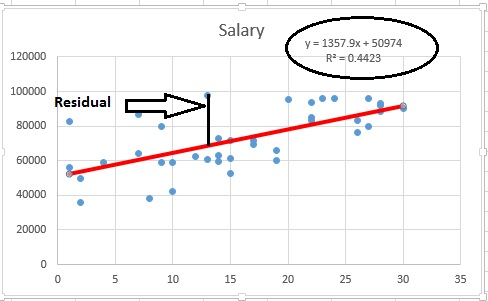You then repeat the process (picking a new line and measuring residuals) until you find the line that results in the lowest overall residual.Once you have it, you get the equation for your line:  y = 1357.9x+50974 (Luckily for us Excel makes the process a lot easier)

Now a quick refresher on the line formula: Y= mX + b (where m = Slope and b = Y-Intercept). This equation is what you would use to make predictions. In our equation a person with 0 years in service would have a salary of 50974: Y = 1357.9(0) + 50974 — Y= 50974. And each year of service would add 1357.90 to the salary.

Before we go start using your equation to start making predictions, we still need to discuss the R² you see below your line equation. I won’t bore you with how R² is calculated. You don’t really need to know how it is calculated to use linear regression, but you do need to know how to read it.

The simplest explanation I can give you for R² is that a value of 1 means perfect fit – every point in your data matches up to your line. 0 on the other hand, means your line doesn’t match anything. Our R² is 0.4423, which really is not that great. I generally prefer to aim for a R² value above 0.6.

How can we improve our R² value? My preference would be to get more data. We currently only have 39 tuples. More data could improve our accuracy. If more data is not available though, you can look at your outliers as Linear Regression can be greatly affected by outliers. Unfortunately outliers are often tricky to deal with. A person with 1 year of service making 100,000 a year would definitely be an outlier, but it is not an impossibility. If this employee is a highly experienced individual who just transferred from another company, it is totally feasible they could be earning 100,000.

The hard truth is, considering only the data we have, we cannot rightfully develop a reliable model. This happens more often than you might think. That is okay though, we will chalk this up as a learning experience and move on.